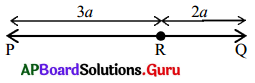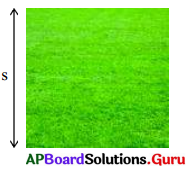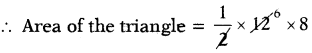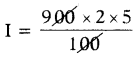# AP Board 7th Class Maths Solutions Chapter 9 Algebraic Expressions Ex 9.4

SCERT AP 7th Class Maths Solutions Pdf Chapter 9 Algebraic Expressions Ex 9.4 Textbook Exercise Questions and Answers.

## AP State Syllabus 7th Class Maths Solutions 9th Lesson Algebraic Expressions Ex 9.4

Question 1.
Find the value of the expression
2x2 – 4x + 5 when
(i) x = 1
(ii) x = – 2
(iii) x = 0.
Given expression is 2x2 – 4x + 5

(i) When x = 1, then
= 2(1)2 – 4(1) + 5
= 2 × 1 – 4 + 5
= 2 – 4 + 5 = 3
When x = 1, then 2x2 – 4x + 5 = 3(ii) When x = – 2, then
= 2(- 2)2 – 4(- 2) + 5
= 2(4) + 8 + 5
= 8 + 8 + 5 = 21
When x = – 2, then 2x2 – 4x + 5 = 21

(iii) When x = 0, then
= 2(0)2 – 4(0) + 5
= 2(0) – 0 + 5
= 0 – 0 + 5 = 5
When x = 0, then 2x2 – 4x + 5 = 5

Question 2.
Find the value of Expressions when m = 2, n = – 1.
(i) 2m + 2n
Given expression is 2m + 2n
If m = 2, n = – 1, then
2m + 2n = 2(2) + 2(- 1) = 4 – 2 = 2
∴ If m = 2, n = – 1, then 2m + 2n = 2

(ii) 3m – n
Given expression is 3m – n
If m = 2, n = – 1, then
3m – n = 3(2) -(-1) = 6 + 1 = 7
∴ If m = 2, n = – 1, then 3m – n = 7

(iii) mn – 2.
Given expression is mn – 2
If m = 2, n = – 1, then
mn – 2 = 2 × (-1)-2
= – 2 – 2 = – 4
∴ If m = 2, n = – 1, then mn – 2 = – 4Question 3.
Simplify and find the value of the expression 5x2 – 4 – 3x2 + 6x + 8 + 5x – 13 when x = – 2.
Given expression is
5x2 – 4 – 3x2 + 6x + 8 + 5x – 13
= (5x2 – 3x2) + (6x + 5x) + (-4 + 8 – 13)
= (5 – 3)x2 + (6 + 5)x + (- 9)
= 2x2 + 11x – 9
If x = – 2, then 2x2 + 11x – 9
= 2(- 2)2 + 11 (- 2) – 9
= 2(4) – 22 – 9
= 8 – 22 – 9
∴ If x = – 2, then 2x2 + 11x – 9 = – 23

Question 4.
Find the length of the line segment PQ when a = 3 cm.From the figure,
Given PR = 3a and RQ = 2a
PQ = PR + RQ
= 3a + 2a
= (3 + 2)a
PQ = 5a
If a = 3 cm, then PQ = 5(3)
∴ PQ = 15 cm

Question 5.
The area of a square field of side ‘s’ meters is s2 sq. m. Find the area of square field, when
(i) s = 5m
(ii) s =12m
(iii) s = 6.5mFrom the figure,
Area of square = s2 sq.m.
(i) If s = 5m, then
s2 = (5)2 = 5 × 5 = 25 sq.m

(ii) If s = 12 m, then
s2 = (12)2 = 12 × 12 = 144 sq.m

(iii) If s = 6.5m, then
s2 = (6.5)2 = 6.5 × 6.5 = 42.25 sq.mQuestion 6.
The area of triangle is given by $$\frac{1}{2}$$ ∙ b ∙ h and if b = 12 cm, h = 8 cm, then find the area of triangle.
Given area of the triangle = $$\frac{1}{2}$$ ∙ b ∙ h
If b = 12 cm, h = 8 cm= 6 × 8 = 48 sq.cm.

Question 7.
Simple interest is given by I = $$\frac{\text { PTR }}{100}$$, If P = ₹ 900, T = 2 years and R = 5%, then find the simple interest.
Given simple interest I = $$\frac{\text { PTR }}{100}$$
If P = ₹ 900, T= 2 and R = 5% then= 9 × 2 × 5 = 90

Question 8.
Find the errors and correct them in the following:
The value of following when a = – 3.
(i) 3 – a = 3 – 3 = 0
3 – a = 3 – (- 3) (when a = – 3)
= 3 + 3 = 6
(Error is – (- 3) = – 3)

(ii) a2 + 3a = (- 3)2 + 3(- 3) = 9 + 0 = 9
a2 + 3a = (- 3)2 + 3(- 3) (when a = – 3)
= (- 3 × – 3) – 9
(Error is 3(- 3) = 0)
= 9 – 9 = 0(iii) a2 – a – 6 = (- 3)2 – (- 3) – 6 = 9 – 3 – 6 = 0
a2 – a – 6
= (- 3)2 – (- 3) – 6 (when a = – 3)
= (- 3 × – 3) + 3 – 6
(Error is – (- 3) = – 3)
= 9 + 3 – 6
= 12 – 6 = 6

(iv) a2 + 4a + 4 = (- 3)2 + 4(-3) + 4 = 9 + 1 + 4 = 14
a2 + 4a + 4
= (- 3)2 + 4(- 3) + 4 (when a = – 3)
= (- 3 × – 3)- 12 + 4
(Error is 4 (- 3) = 1)
= 9 – 12 + 4 = 13 – 12 = 1

(v) a3 – a2 – 3 = (- 3)3 – (-3)2 – 3 = – 9 + 6 – 3 = – 6